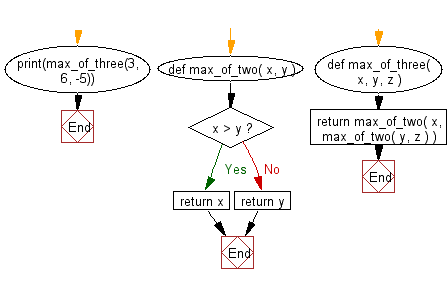﻿ Python Exercise: Find the Max of three numbers - w3resource# Python Exercise: Find the Max of three numbers

## Python Functions: Exercise-1 with Solution

Write a Python function to find the Max of three numbers.

Sample Solution:-

Python Code:

``````def max_of_two( x, y ):
if x > y:
return x
return y
def max_of_three( x, y, z ):
return max_of_two( x, max_of_two( y, z ) )
print(max_of_three(3, 6, -5))
```
```

Sample Output:

```6
```

Pictorial presentation:Flowchart:## Visualize Python code execution:

The following tool visualize what the computer is doing step-by-step as it executes the said program:

Python Code Editor:

Have another way to solve this solution? Contribute your code (and comments) through Disqus.

What is the difficulty level of this exercise?

Test your Programming skills with w3resource's quiz.

﻿

## Python: Tips of the Day

Decapitalizes the first letter of a string:

Example:

```def tips_decapitalize(s, upper_rest=False):
return s[:1].lower() + (s[1:].upper() if upper_rest else s[1:])
print(tips_decapitalize('PythonTips'))
print(tips_decapitalize('PythonTips', True))
```

Output:

```pythonTips
pYTHONTIPS
```